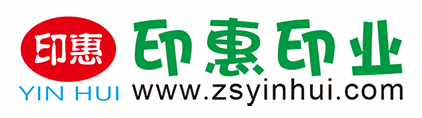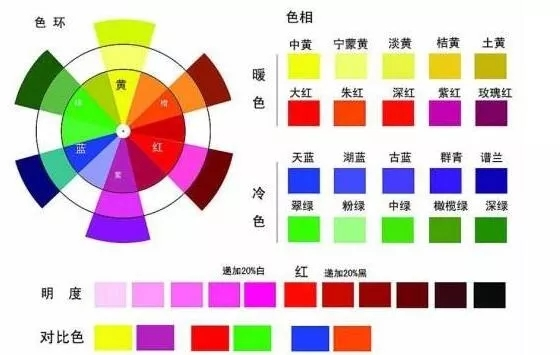#24小时咨询热线 13420456122

## 业内常识

##### 业内常识

 丝印调色表 作者：admin 访问量：5823 日期：2018/10/12 9:34:10丝印油墨调色时的基本色的调和: 红加黄变橙，红加蓝变紫，黄加蓝变绿。红、黄、蓝是三原色，橙、紫、绿则是三间色。间色与间色相调合就会变成各类灰色。但灰色都应该是有色彩倾向的，譬如：蓝灰，紫灰，黄灰等。 1.红加黄变橙 2.少黄多红变深橙 3.少红多黄变浅黄 4.红加蓝变紫 5.少蓝多红变紫再加多红变玫瑰红 6.黄加蓝变绿 7.少黄多蓝变深蓝 8.少蓝多黄变浅绿 9.红加黄加少蓝变棕色 10.红加黄加蓝变灰黑色（按分量多少调可调出多种深浅不一的颜色） 11.红加蓝变紫再加白变浅紫 12.黄加少红变深黄加白变土黄 13.黄加少红变深黄 14.黄加蓝变绿加白变奶绿 15.红加黄加少蓝加白变浅棕 16.红加黄加蓝变灰黑色加多白变浅灰 17.黄加蓝变绿加蓝变蓝绿 18.红加蓝变紫再加红加白变粉紫红（玫瑰） 19.少红加白变粉红 红、黄、蓝是三原色，橙、紫、绿则是三间色。间色与间色相调合就会变成各类灰色。 朱红色 + 黑色少量 = 啡色 天蓝色 + 黄色 = 草绿、 嫩绿 天蓝色 + 黑色 + 紫 = 浅蓝紫 草绿色 + 少量黑色 = 墨绿 天蓝色 + 黑色 = 浅灰蓝 天蓝色 + 草绿色 = 蓝绿 白色 + 红色 + 黑色少量 = 禇石红 天蓝色 + 黑色（少量） = 墨蓝 白色 + 黄色 + 黑色 = 熟褐 玫红色 + 黑色（少量） = 暗红 红色 + 黄 + 白 = 人物的皮肤颜色 玫红色 + 白色 = 粉玫红 蓝色 + 白色 = 粉蓝 黄色 + 白色 = 米黄 玫红色 + 黄色 = 大红 ( 朱红、桔黄、藤黄 ) 朱红色 + 黑色少量 = 啡色 天蓝色 + 黄色 = 草绿、嫩绿 天蓝色 + 黑色 + 紫 = 浅蓝紫 草绿色 + 少量黑色 = 墨绿 天蓝色 + 黑色 = 浅灰蓝 天蓝色 + 草绿色 = 蓝绿 白色 + 红色 + 黑色少量 = 禇石红 天蓝色 + 黑色（少量） = 墨蓝 白色 + 黄色 + 黑色 = 熟褐 玫红色 + 黑色（少量） = 暗红 红色 + 黄 + 白 = 人物的皮肤颜色 玫红色 + 白色 = 粉玫红 蓝色 + 白色 = 粉蓝 黄色 + 白色 = 米黄 粉柠檬黄 = 柠檬黄 + 纯白色 藤黄 色 = 柠檬黄 + 玫瑰红 桔黄 色 = 柠檬黄 + 玫瑰红 土黄 色 = 柠檬黄 + 纯黑色 + 玫瑰红 熟褐 色 = 柠檬黄 + 纯黑色 + 玫瑰红 粉玫瑰红 = 纯白色 + 玫瑰红 朱红 色 = 柠檬黄 + 玫瑰红 暗红 色 = 玫瑰红 + 纯黑色 紫红 色 = 纯紫色 + 玫瑰红 褚石 红 = 玫瑰红 + 柠檬黄 + 纯黑色 粉蓝 色 = 纯白色 + 天蓝色 蓝绿 色 = 草绿色 + 天蓝色 灰蓝 色 = 天蓝色 + 纯黑色 浅灰 蓝 = 天蓝色 + 纯黑色 + 纯紫色 粉绿 色 = 纯白色 + 草绿色 黄绿 色 = 柠檬黄 + 草绿色 墨绿 色 = 草绿色 + 纯黑色 粉紫 色 = 纯白色 + 纯紫色 啡色 = 玫瑰红 + 纯黑色
• 返回顶部
• 13420456122
• 24小时咨询热线
• zsyinhui@163.com
• 在线咨询
• 微信二维码